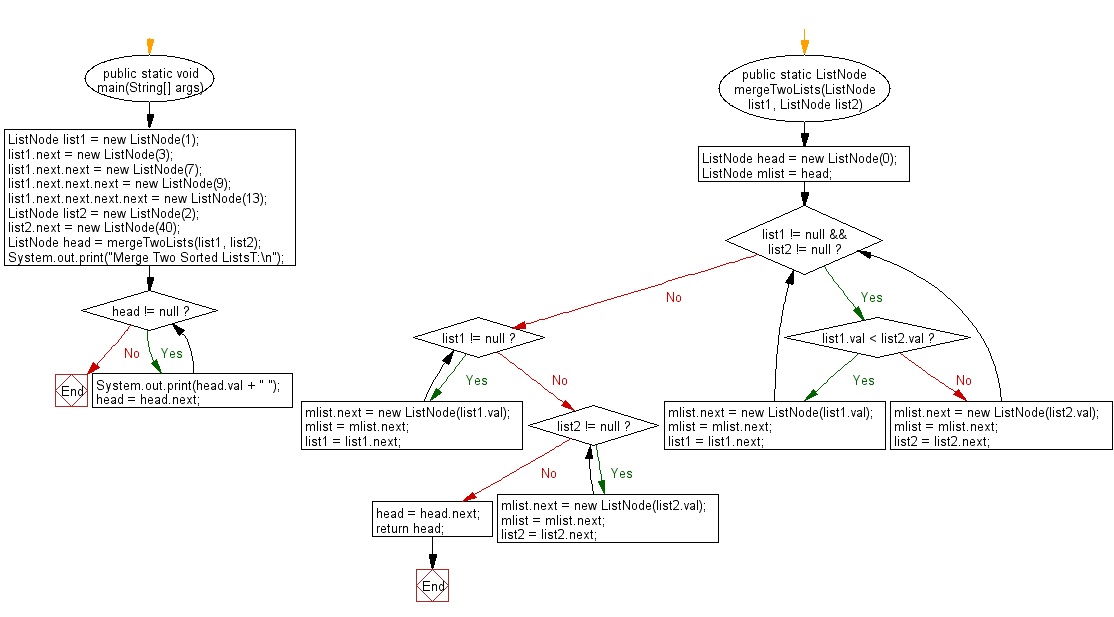﻿ Java exercises: Merge two given sorted lists - w3resource# Java Exercises: Merge two given sorted lists

## Java Basic: Exercise-143 with Solution

Write a Java program to merge two given sorted lists.

Sample Solution:

Java Code:

``````public class Solution {
public static void main(String[] args) {
ListNode list1 = new ListNode(1);
list1.next = new ListNode(3);
list1.next.next = new ListNode(7);
list1.next.next.next = new ListNode(9);
list1.next.next.next.next = new ListNode(13);
ListNode list2 = new ListNode(2);
list2.next = new ListNode(40);
System.out.print("Merge Two Sorted ListsT:\n");
}
}

public static ListNode mergeTwoLists(ListNode list1, ListNode list2) {
while (list1 != null && list2 != null) {
if (list1.val < list2.val) {
mlist.next = new ListNode(list1.val);
mlist = mlist.next;
list1 = list1.next;
} else {
mlist.next = new ListNode(list2.val);
mlist = mlist.next;
list2 = list2.next;
}
}
while (list1 != null) {
mlist.next = new ListNode(list1.val);
mlist = mlist.next;
list1 = list1.next;
}
while (list2 != null) {
mlist.next = new ListNode(list2.val);
mlist = mlist.next;
list2 = list2.next;
}

}
}

class ListNode {
int val;
ListNode next;

ListNode(int val) {
this.val = val;
this.next = null;
}
}
```
```

Sample Output:

```Merge Two Sorted ListsT:
1 2 3 7 9 13 40
```

Flowchart:Java Code Editor:

Company:

What is the difficulty level of this exercise?

Test your Programming skills with w3resource's quiz.

﻿

## Java: Tips of the Day

countOccurrences

Counts the occurrences of a value in an array.

Use Arrays.stream().filter().count() to count total number of values that equals the specified value.

```public static long countOccurrences(int[] numbers, int value) {
return Arrays.stream(numbers)
.filter(number -> number == value)
.count();
}
```

Ref: https://bit.ly/3kCAgLb